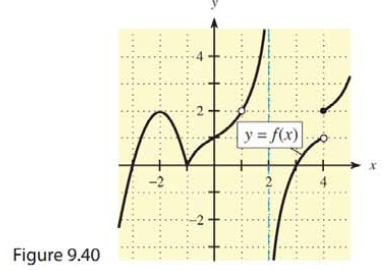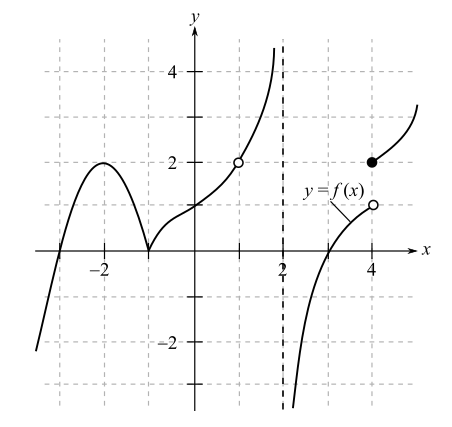Chapter 9, Problem 46RE### Mathematical Applications for the ...

11th Edition
Ronald J. Harshbarger + 1 other
ISBN: 9781305108042

#### Solutions

Chapter
Section### Mathematical Applications for the ...

11th Edition
Ronald J. Harshbarger + 1 other
ISBN: 9781305108042
Textbook Problem

# Use the graph of y = f(x) in Figure 9.40 on the previous page to answer the questions in Problems 44-46.Is f(x) differentiable at ( a )   x   =   − 2 ? ( b )   x = 2 ?

(a)

To determine

Whether the function with the following graph is differentiable at x=2,Explanation

Given Information:

The provided graph of the function is,

Explanation:

Consider the provided graph of function,

A function is differentiable on a point, when the function is continuous at the point, there is no sharp corner at the point and no vertical tangent line can be drawn through the point...

(b)

To determine

Whether the function with the following graph is differentiable at x=2,### Still sussing out bartleby?

Check out a sample textbook solution.

See a sample solution

#### The Solution to Your Study Problems

Bartleby provides explanations to thousands of textbook problems written by our experts, many with advanced degrees!

Get Started

#### Prove that limx0+lnx=

Single Variable Calculus: Early Transcendentals, Volume I

#### In Exercises 69-74, rationalize the numerator. 69. 2x3

Applied Calculus for the Managerial, Life, and Social Sciences: A Brief Approach

#### For what values of p does the series converge?

Study Guide for Stewart's Single Variable Calculus: Early Transcendentals, 8th

#### The graph at the right has equation:

Study Guide for Stewart's Multivariable Calculus, 8th